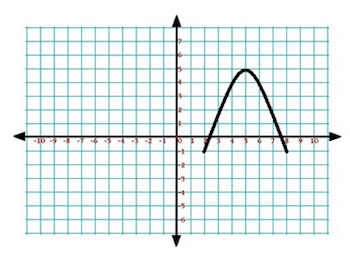# Find the Maximum Value of a Function: Practice & Overview

An error occurred trying to load this video.

Try refreshing the page, or contact customer support.

Coming up next: What is a Matrix?

### You're on a roll. Keep up the good work!

Replay
Your next lesson will play in 10 seconds
• 0:00 What Is Maximum Value?
• 0:27 How to Determine Maximum Value
• 2:56 Real World Examples
• 4:43 Lesson Summary

Want to watch this again later?

Timeline
Autoplay
Autoplay
Speed

#### Recommended Lessons and Courses for You

Lesson Transcript
Instructor: Jennifer Beddoe
The maximum value of a function can be found at its highest point, or vertex, on a graph. This lesson will show you how to find the maximum value of a function and give some examples. A quiz will complete the lesson.

## What Is Maximum Value?

The maximum value of a function is the place where a function reaches its highest point, or vertex, on a graph. For instance, in this image, the maximum value of the function is y equals 5.Practically, finding the maximum value of a function can be used to determine maximum profit or maximum area. It can be very helpful in running a profitable business or any architecture or building project.

## How to Determine Maximum Value

There are three methods for determining the maximum value of a quadratic equation. Each of them can be used in their own unique setting to determine the maximum.

The first way is graphing. You can find the maximum value visually by graphing the equation and finding the maximum point on the graph. This is especially easy when you have a graphing calculator that can do the work for you. Again, using this graph, you can see that the maximum point of the graph is at y = 5.

The second way to determine the maximum value is using the equation y = ax2 + bx + c.

If your equation is in the form ax2 + bx + c, you can find the maximum by using the equation:

max = c - (b2 / 4a).

The first step is to determine whether your equation gives a maximum or minimum. This can be done by looking at the x2 term. If this term is positive, the vertex point will be a minimum. If it is negative, the vertex will be a maximum.

After determining that you actually will have a maximum point, use the equation to find it. For example, let's find the maximum point of:

-x2 + 4x - 2.

Since the term with the x2 is negative, you know there will be a maximum point. To find it, plug the values into the equation:

max = c - (b2 / 4a)

This will give us:

max = -2 - (42 / 4 * (-1))

4 squared is 16, and 4 times -1 is -4. 16 divided by -4 is -4. And, -2 minus -4 becomes -2 plus 4 because two negatives become a positive, so we end up with a maximum value of 2.

There's one more way to determine the maximum value of a function, and that is from the equation:

y = a(x - h)2 + k

As with the last equation, the a term in this equation must be negative for there to be a maximum. If the a term is negative, the maximum can be found at k. No equation or calculation is necessary - the answer is just k.

For example, let's find the maximum of the equation:

-3(x - 5)2 - 7

Since the a term is -3, there will be a maximum at -7.

## Real World Examples

Now let's look at a few examples of how this information is helpful in the real world.

To unlock this lesson you must be a Study.com Member.

### Register to view this lesson

Are you a student or a teacher?

### Unlock Your Education

#### See for yourself why 30 million people use Study.com

##### Become a Study.com member and start learning now.
Back
What teachers are saying about Study.com

### Earning College Credit

Did you know… We have over 160 college courses that prepare you to earn credit by exam that is accepted by over 1,500 colleges and universities. You can test out of the first two years of college and save thousands off your degree. Anyone can earn credit-by-exam regardless of age or education level.